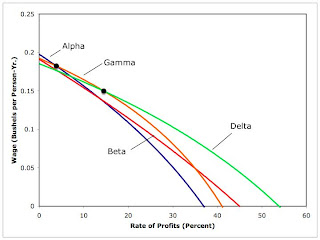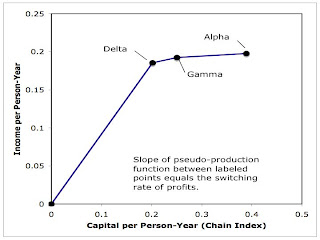## Wednesday, May 20, 2009

### A Neoclassical Response To The Cambridge Capital Controversy

1.0 Introduction
Around 1980, Edwin Burmeister could have justly thought that he was expressing the most prominent neoclassical response to the Cambridge Capital Controversy. He had championed David Champernowne's chain index as a defense of the aggregate neoclassical model, and continued to do so. Nowadays, though, mainstream economists make claims based on the aggregate model apparently in complete ignorance that they had ever been competently challenged:
"However, the damage had been done, and Cambridge, UK, 'declared victory': Levhari was wrong, Samuelson was wrong, Solow was wrong, MIT was wrong and therefore neoclassic economics was wrong. As a result there are some groups of economists who have abandoned neoclassical economics for their own refinements of classical economics. In the United States, on the other hand, mainstream economics goes on as if the controversy had never occurred." -- Edwin Burmeister (2000)
This post illustrates, by means of an example, elements of Burmeister's approach to the neoclassical aggregate model. It is exposition, with next to no criticism.

2.0 Technology
Consider a very simple economy in which a single consumption good, corn, is produced from inputs of labor, iron, and (seed) corn. All production processes in this example require a year to complete. Two production processes are known for producing corn, and two processes are known for producing iron. These processes require inputs to be available at the beginning of the year for each unit output produced and available at the end of the year. Each corn-producing process produces one bushel corn at the scale of operations shown in Table 1. Similarly, each iron-producing process produces one ton iron at the scale shown in Table 1.
 Inputs Corn Industry Iron Industry A B C D Labor (Person-Years): 2 3 1 2 Iron (Tons): 3/50 1/10 3/5 1/2 Corn (Bushels): 1/2 1/4 1/2 3/5 Output (Various): 1 1 1 1
Apparently, inputs of iron and corn can be traded off in producing corn outputs. The process that requires more iron also requires more labor. Inputs of iron and corn are also traded off in producing iron. But in iron production, the process requiring a greater quantity of iron input requires less labor.

A technique consists of a process for producing iron and a process for producing corn. Thus, there are four techniques in this example. They are defined in Table 2.
 Technique Processes Alpha A, C Beta A, D Gamma B, C Delta B, D

3.0 Quantity Flows
Suppose firms have adopted the alpha technique and they produce 20/43 bushels corn with process A and 3/43 tons iron with process C. One can see, from Table 1, that these firms will employ 40/43 person-years in the corn industry and 3/43 person-years in the iron industry - that is, a total of one person-year throughout the economy. Likewise, firms in the corn industry will purchase inputs of 6/215 tons iron, while firms in the iron industry will purchase inputs of 9/215 tons iron in the iron industry, for a total of 3/43 tons iron inputs throughout the economy. The produced iron at the end of the year exactly replaces the iron used as input, leaving a net output of 17/86 bushels corn. (Calculating corn inputs in the two industries is left as an exercise for the reader.)

Since these processes can be equally scaled up to any desired level, I have described a stationary economy on a per person-year basis. Table 3 shows the results of these calculations, as well as similar calculations for the gamma and delta technique. The beta technique is never cost-minimizing and is not shown in Table 3.
 Technique Variable Value Alpha Gross Outputs (3/43 Tons, 20/43 Bushels) Capital Goods (3/43 Tons, 23/86 Bushels) Net Output 17/86 Bushels Corn Gamma Gross Outputs (1/13 Tons, 4/13 Bushels) Capital Goods (1/13 Tons, 3/26 Bushels) Net Output 5/26 Bushels Corn Delta Gross Outputs (1/17 Tons, 5/17 Bushels) Capital Goods (1/17 Tons, 37/340 Bushels) Net Output 63/340 Bushels Corn

4.0 Prices
In a steady state, the same rate of profits is earned on all processes in use. Furthermore, charging that rate of profits on a process not eligible for use results in costs in that process exceeding the revenues. That is, we seek steady state prices corresponding with the cost-minimizing technique.

Suppose the alpha technique is cost minimizing. Prices for the iron-producing process (C) must satisfy the following equation:
(3/5 pα + 1/2)(1 + r) + wα = pα,
where pα is the price of iron, wα is the wage, and r is the rate of profits. The wage is paid at the end of the year, and corn is taken as the numeraire (so the price of a bushel corn is unity). Likewise, prices for the corn producing process (A) satisfy the following equation:
(3/50 pα + 1/2)(1 + r) + 2 wα = 1

I have specified a system of two equations in three variables. The wage and price of iron can be found as a function of the third variable, that is, the rate of profits. Table 4 displays this solution, as well as the solutions for the corresponding systems of equations for the other three techniques.

 Technique Variable Equation Alpha Wage wα(r) = (27 r2 - 56 r + 17)/[2 (43 - 57 r)] Price of Iron pα(r) = 25 (3 + r)/(43 - 57 r) Beta Wage wβ(r) = (107 r2 - 286 r + 107)/[40 (14 - 11 r)] Price of Iron pβ(r) = 5 (11 +r)/[2 (14 - 11 r)] Gamma Wage wγ(r) = (2 r2 - 13 r + 5)/[2 (13 - 17 r)] Price of Iron pγ(r) = 5(9 + 5 r)/[2 (13 - 17 r)] Delta Wage wδ(r) = (13 r - 7)(r - 9)/[20 (17 - 13 r)] Price of Iron pδ(r) = (33 + 13 r)/(17 - 13 r)

Figure 1 graphs the wage-rate of profits curves for each technique. The cost-minimizing technique corresponds to the curve on the outer envelope. The wage-rate of profits curves for the alpha, gamma, and delta technique comprise the wage-rate of profits frontier. Alpha is cost-minimizing at low rate of profits, delta is cost-minimizing at high rates, and gamma is cost-minimizing at intermediate rates. Notice that for each pair of techniques, the wage-rate of profits curves cross at most once in the first quadrant. There is no reswitching, either on or off the frontier, in this example.Figure 1: Wage-Rate of Profits Frontier

5.0 Champernowne's Chain Index

The above analysis specifies for each rate of profits (or for each wage) which technique will be adopted by cost minimizing firms. At switch points, linear combinations of techniques are cost-minimizing. The above analysis also determines the price of each capital good (e.g. corn and iron) for each rate of profits, as well as the composition of capital goods used in each technique per person-year. Figure 2 can thus be drawn based on this analysis.Figure 2: Value of Capital and the Rate of Profits

Figure 2 shows the effects of both real and price Wicksell effects. The two horizontal lines arise from switch points. At switch points the composition of capital goods varies with the technique, while the rate of profits and the prices of capital goods are fixed. In other words, "real" capital varies in some sense. So the horizontal lines show real Wicksell effects. The curved, non-horizontal, segments display price Wicksell effects. That is, at non-switching points, the composition of capital goods remains invariant, but the prices of capital goods vary. Consequently, the numeraire value of the basket of capital goods varies here also.

Champernowne's chain index (Figure 3) sums up real Wicksell effects alone. Price Wicksell effects are abstracted from. The value of capital goods at the rate of profits of zero is taken in Figure 3 from Figure 2. Horizontal lines are drawn in Figure 3 at the same rates of profits at which they appear in Figure 2. The horizontal lines are also the same length. Vertical lines are drawn between horizontal lines.Figure 3: Chain Index Value of Capital and the Rate of Profits

Champernowne's chain index only makes sense of the neoclassical parable in this case because all steps in Figure 3 slope down to the right. In other words, for an infinitesimal variation of the rate of profits around a switch point, the capital intensity of the cost-minimizing technique at the lower rate of profits exceeds the capital intensity of the cost-minimizing technique at the higher rate of profits. That is, Burmeister's defense of the neoclassical parable only applies in cases in which real Wicksell effects happen to be always negative:
"It follows, then, that a negative real Wicksell effect is the appropriate concept of 'capital deepening' in a model with many heterogeneous capital goods... Imposing some set of conditions on the technology ... should be sufficient to assure that the real Wicksell effect is always negative. Such conditions would be of interest - especially if they could be empirically tested - since they would validate the qualitative conclusions derived from one-good models often used in macroeconomics without any theoretical justification... Unfortunately, no set of such sufficient conditions is known, but the literature on capital aggregation suggests that they would impose severe restrictions on the technology." -- Edwin Burmeister (1987)

6.0 A Pseudo-Production Function
I finally turn to the aggregate neoclassical production function used in the neoclassical parable:
Y = F(K, L),
where Y is net income, K is capital, and L is labor. Since Constant Returns to Scale are assumed, one can divide through by the labor input:
Y/L = F(K/L, 1)
Or:
y = f(k),
where y is net output per worker and k is capital per worker, in some sense. Figure 4 graphs this function for the example, where Champernowne's chain index is used to measure capital per worker. (If net output consisted of more than the numeraire good, a chain index would be used to measure output also.)Figure 4: Pseudo-Production Function for the Example

Using this construction, the equilibrium condition that the rate of profits equal the marginal product of capital holds at switch points:
r = f ' (k)
This analysis has accepted that the value of capital goods (that is, the "quantity of capital") depends on the rate of profits. Recall, however, that this analysis only applies to examples in which real Wicksell effects happen to be always negative.

References
• Salvatore Baldone (1984). "From Surrogate to Pseudo Production Functions", Cambridge Journal of Economics, V. 8: 271-288
• Edwin Burmeister (1980) Capital Theory and Dynamics, Cambridge University Press
• Edwin Burmeister (1987) "Wicksell Effects", in The New Palgrave, (ed. by J. Eatwell, M. Milgate, and P. Newman), Macmillan
• Edwin Burmeister (2000). "The Capital Theory Controversy" in Critical Essays on Piero Sraffa's Legacy in Economics (ed. by H. D. Kurz), Cambridge University Press
• D. G. Champernowne (1953-1954). "The Production Function and the Theory of Capital: A Comment", Review of Economic Studies, V. 21: 112-135

#### 1 comment:Anonymous said...

«In the United States, on the other hand, mainstream economics goes on as if the controversy had never occurred.»

And that is as it should be: because if the truth is un-American, then too bad for the truth.

The central verity of neoclassical economics, that income is directly determined by productivity, is what American economics is all about, and useless concerns with theoretical correctness are just un-American.

:-)